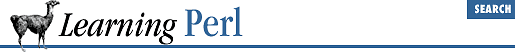#Chapter 9Miscellaneous Control Structures## 9.5 Expression Modifiers

As Yet Another Way to indicate "if this, then that," Perl allows you to tag an if modifier onto an expression that is a standalone statement, like this:

``some_expression` if `control_expression`;`

In this case, `control_expression` is evaluated first for its truth value (using the same rules as always), and if true, `some_expression` is evaluated next. This is roughly equivalent to

```if (`control_expression`) {
`some_expression`;
}```

except that you don't need the extra punctuation, the statement reads backwards, and the expression must be a simple expression (not a block of statements). Many times, however, this inverted description turns out to be the most natural way to state the problem. For example, here's how you can exit from a loop when a certain condition arises:

```LINE: while (<STDIN>) {
last LINE if /^From: /;
}```

See how much easier that is to write? And you can even read it in a normal English way: "last line if it begins with From."

Other parallel forms include the following:

````exp2` unless `exp1`; # like: unless (`exp1`) { `exp2`; }
`exp2` while `exp1`;  # like: while (`exp1`) { `exp2`; }
`exp2` until `exp1`;  # like: until (`exp1`) { `exp2`; }```

All of these forms evaluate `exp1` first, and based on that, do or don't do something with `exp2`.

For example, here's how to find the first power of two greater than a given number:

```chomp(\$n = <STDIN>);
\$i = 1;                 # initial guess
\$i *= 2 until \$i > \$n;  # iterate until we find it```

Once again, we gain some clarity and reduce the clutter.

These forms don't nest: you can't say `exp3` `while` `exp2` `if` `exp1`. This is because the form `exp2` `if` `exp1` is no longer an expression, but a full-blown statement, and you can't tack one of these modifiers on after a statement.